# DC vs Marvel Comic Universe: which movies are doing better?

July 16, 2017
By

Want to share your content on R-bloggers? click here if you have a blog, or here if you don't.

The latest Spider-man movie, Spider-man: Homecoming, has high hopes for the Marvel Comic Universe just as Wonder woman from the DC universe proved a huge success. The Marvel and DC comic universes both have some great superheros, but only a few of them are succeeding at the box office. I wanted to compare these super-hero movies and their success at the box office using R.

Marvel vs DC? It’s on. As close as we can get seeing Spider-man against Batman.

Let’s get started.

 ```1 2 3 4 5 6 7 8 9 10 11 12 ``` ```# To get data from Wikipedia library(rvest) # To manipulate data library(dplyr) # To plot library(ggplot2) # Make plots look good library(ggthemes) # Apply lables library(directlabels) # Better labeling library(scales)```

# Get the Marvel and DC comic movies data

Thankfully, Wikipedia already lists the movie data for us. The challenge was to get it scraped. The package `rvest` makes it very easy. You just need to find the right element. In Chrome, you right click on the table and select `Inspect`. Once you get to the element you want to scrape, you right-click on the HTML markup and select `Copy` then `Copy XPath`.

 ```1 2 3 4 5 6 7 8 9 ``` ```marvel_movies_data <- read_html('https://en.wikipedia.org/wiki/List_of_films_based_on_Marvel_Comics') %>% html_node(xpath = '//*[@id="mw-content-text"]/div/table') %>% html_table(fill = TRUE, trim = TRUE) %>% setNames(., nm = paste0("X", 1:8)) %>% slice(4:n()-2) %>% mutate_at(.cols = 4:8, .funs = funs(as.numeric(gsub(pattern = '[\$,]', replacement = '', x = .)))) %>% mutate(release_date = as.Date(X3, format = '%B %d, %Y'), movie = X1, universe = 'marvel', budget = X4*10^6, opening_wkend = X5, worldwide_gross = X8)```

Here are the details line by line:

2. Select the table using the copied `XPath`
3. Set some parameters to take care of merged cells
4. Rename all columns to X1 to X8
5. Filter out the first three rows and the last two rows
6. Remove the dollar sign and commas from the numbers
7. Change the format of the date and rename a few columns

Do the same thing for the DC movies data.

 ```1 2 3 4 5 6 7 8 9 ``` ```dc_movies_data <- read_html('https://en.wikipedia.org/wiki/List_of_films_based_on_DC_Comics') %>% html_node(xpath = '//*[@id="mw-content-text"]/div/table') %>% html_table(fill = TRUE, trim = TRUE) %>% setNames(., nm = paste0("X", 1:7)) %>% slice(3:n()-1) %>% mutate_at(.cols = 4:7, .funs = funs(as.numeric(gsub(pattern = '[\$,]', replacement = '', x = .)))) %>% mutate(release_date = as.Date(X3, format = '%B %d, %Y'), movie = X1, universe = 'dc', budget = X4*10^6, opening_wkend = X5, worldwide_gross = X7)```

# Manipulate the data further

Combine the Marvel and DC comic universe data, but select only a few columns. Also calculate the profits and the profit ratio.

 ```1 2 ``` ```marvel_dc <- bind_rows(select(marvel_movies_data, universe, movie, release_date, budget, opening_wkend, worldwide_gross), select(dc_movies_data, universe, movie, release_date, budget, opening_wkend, worldwide_gross)) %>% mutate(profit = worldwide_gross - budget, profit_ratio = worldwide_gross/budget)```

Find out the top movies by profit, total box office, and expense. We will leave Spider-man in for comparison.

 ```1 2 3 4 ``` ```highest_profit_ratio <- group_by(marvel_dc, universe) %>% top_n( n = 1, wt = profit_ratio) %>% bind_rows(., filter(marvel_dc, movie == 'Spider Man: Homecoming')) highest_grossing <- group_by(marvel_dc, universe) %>% top_n( n = 1, wt = worldwide_gross) %>% bind_rows(., filter(marvel_dc, movie == 'Spider Man: Homecoming')) most_expensive <- group_by(marvel_dc, universe) %>% top_n( n = 1, wt = budget) %>% bind_rows(., filter(marvel_dc, movie == 'Spider Man: Homecoming')) most_profitable <- group_by(marvel_dc, universe) %>% top_n( n = 1, wt = profit) %>% bind_rows(., filter(marvel_dc, movie == 'Spider Man: Homecoming'))```

# Let’s start plotting

## Worldwide Gross at the Box Office

First, let’s look at the box office result of these movies.

 ```1 2 3 4 5 6 ``` ```g <- ggplot(data = marvel_dc, aes(x = release_date, y = worldwide_gross, group = universe, color = universe)) + geom_point() + scale_y_continuous(labels = dollar) + theme_fivethirtyeight() g <- g + ylab("Worldwide Gross") + xlab(label = "Release Date") + scale_color_manual(values = c("dc" = "#0066A9", "marvel" = "red")) g <- g + geom_text(data = highest_grossing, aes(x = release_date, y = worldwide_gross, label = movie, color = universe), vjust = -0.5, show.legend = FALSE) g <- g + labs(title = "Worldwide Gross at the Box Office", subtitle = "All current dollars", caption = "Wikipedia data") g <- g + theme(legend.position = "none") g```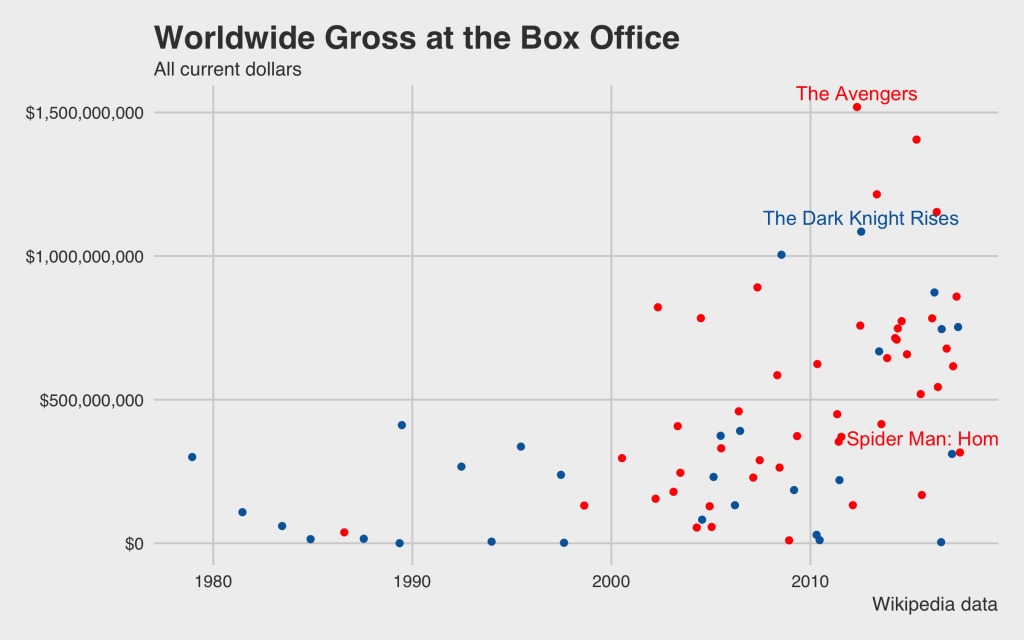From the Marvel universe, The Avengers scored huge, collecting over \$1.5B worldwide. The Dark Knight Rises, the everybody’s favorite Batman movie, collected slightly over \$1B. After 2006 or so, it also looks like that the Marvel comic-heroes started bringing more money in the box office.

## Budget of the Movies

Next, let’s see the budget of these movies.

 ```1 2 3 4 5 6 ``` ```g <- ggplot(data = marvel_dc, aes(x = release_date, y = budget, group = universe, color = universe)) + geom_point() + scale_y_continuous(labels = dollar) + theme_fivethirtyeight() g <- g + scale_color_manual(values = c("dc" = "#0066A9", "marvel" = "red")) g <- g + geom_text(data = most_expensive, aes(x = release_date, y = budget, label = movie, color = universe), vjust = -0.5, show.legend = FALSE) g <- g + labs(title = "Budget of the Movies", subtitle = "All current dollars", caption = "Wikipedia data") g <- g + theme(legend.position = "none") g```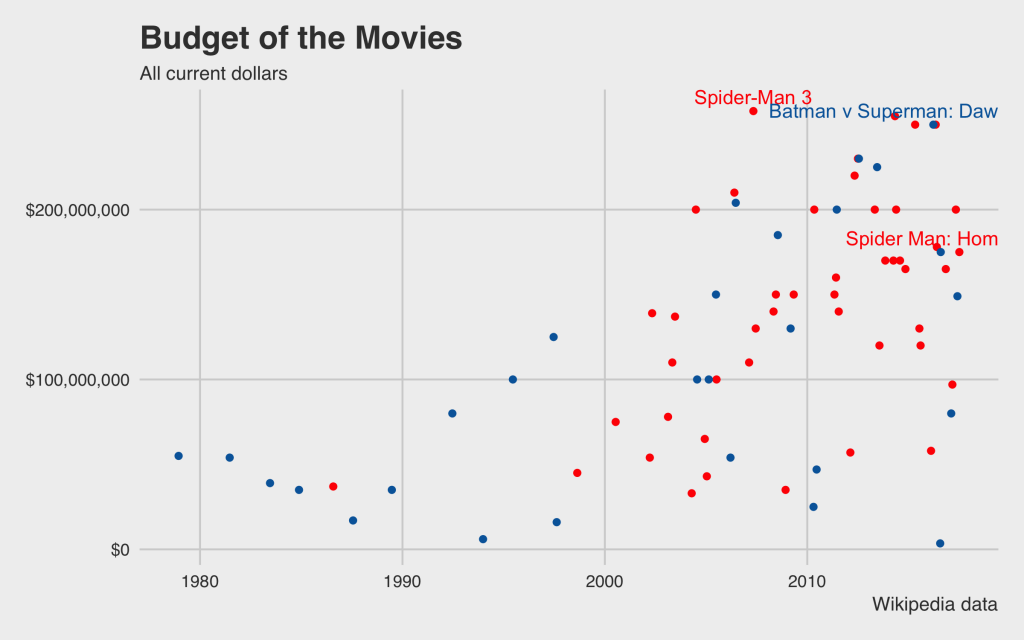Majority of these movies are over \$100M and again you see an upward trend after the early 2000s.

## Budget vs Worldwide Gross

So, if there was an uptick in the budget and we saw more money coming in, is there a correlation between budget and worldwide gross? Let’s see:

 ```1 2 3 4 5 6 ``` ```g <- ggplot(data = marvel_dc, aes(x = budget, y = worldwide_gross, group = universe, color = universe)) + geom_point() + scale_y_continuous(labels = dollar) + scale_x_continuous(labels = dollar) + theme_fivethirtyeight() g <- g + scale_color_manual(values = c("dc" = "#0066A9", "marvel" = "red")) #g <- g + geom_text(data = most_expensive, aes(x = release_date, y = budget, label = movie, color = universe), vjust = -0.5, show.legend = FALSE) g <- g + labs(title = "Budget and Worldwide Gross", subtitle = "All current dollars", caption = "Wikipedia data") g <- g + theme(legend.position = "none") g```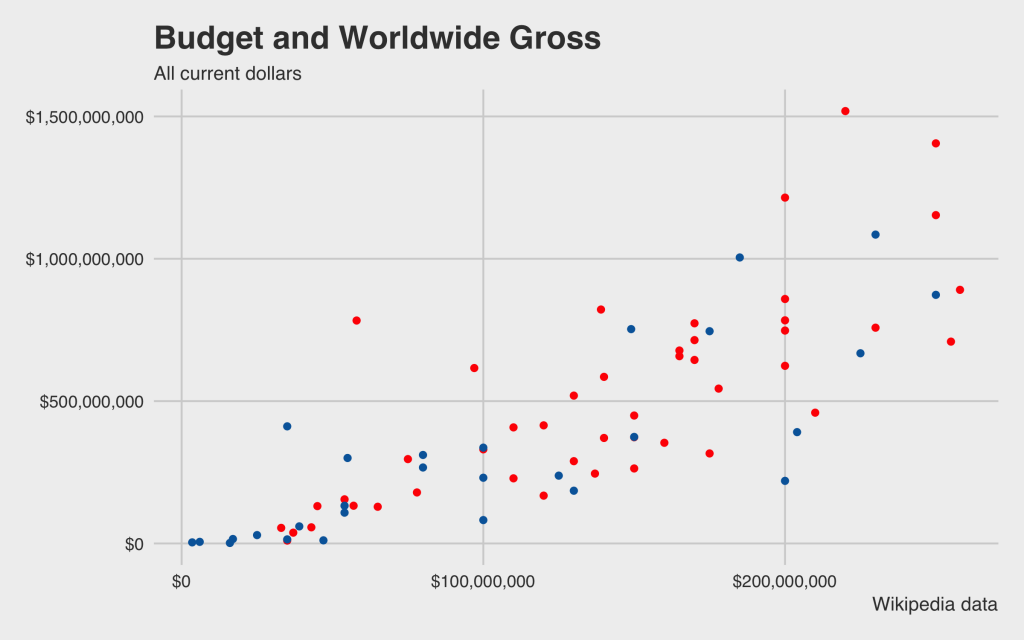You can see definitely see a positive trend.

## Profits

Now let’s look at the profits of these movies.

 ```1 2 3 4 5 6 ``` ```g <- ggplot(data = marvel_dc, aes(x = release_date, y = profit, group = universe, color = universe)) + geom_point() + scale_y_continuous(labels = dollar) + theme_fivethirtyeight() g <- g + scale_color_manual(values = c("dc" = "#0066A9", "marvel" = "red")) g <- g + geom_text(data = most_profitable, aes(x = release_date, y = profit, label = movie, color = universe), vjust = -0.5, show.legend = FALSE) g <- g + labs(title = "Profit from the Movies", subtitle = "All current dollars", caption = "Wikipedia data") g <- g + theme(legend.position = "none") g```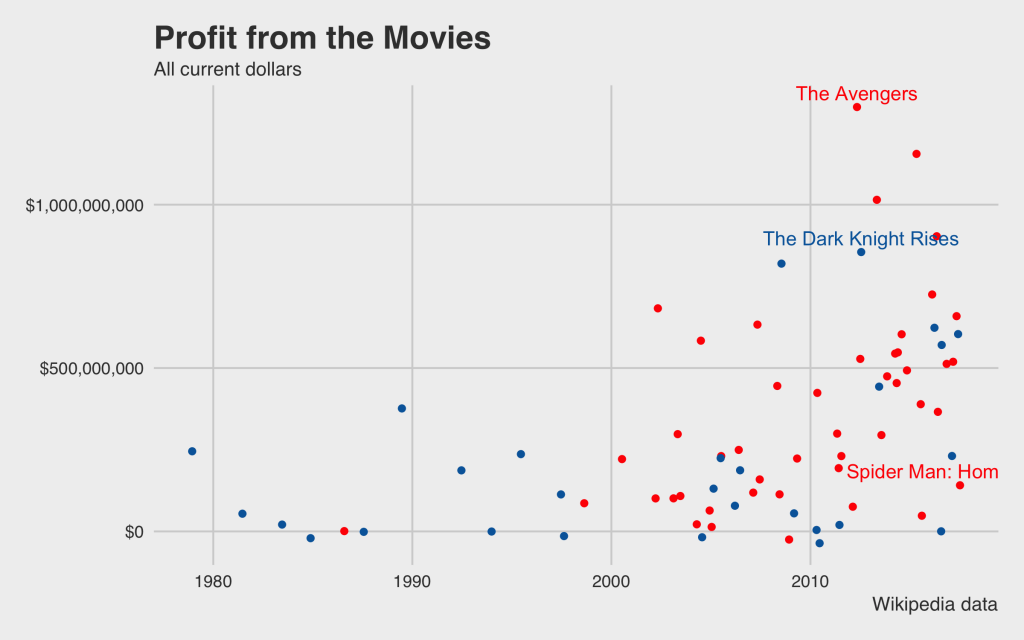The Avengers and the Dark Knight are still the most profitable for the both the universes. Whereas, the Spider-man lags behind.

## Profit Ratio

Pure profit doesn’t show the ratio of revenue over expenses. Let’s take a look at the profit ratio instead.

 ```1 2 3 4 5 6 7 ``` ```g <- ggplot(data = marvel_dc, aes(x = release_date, y = profit_ratio, group = universe, color = universe)) + geom_point() + scale_y_continuous() + theme_fivethirtyeight() g <- g + scale_color_manual(values = c("dc" = "#0066A9", "marvel" = "red")) g <- g + geom_text(data = highest_profit_ratio, aes(x = release_date, y = profit_ratio, label = movie, color = universe), vjust = -0.5, show.legend = FALSE) g <- g + labs(title = "Profit Ratio of the Movies", subtitle = "Profit/Budget. Using all current dollars", caption = "Wikipedia data") g <- g + theme(legend.position = "none") g <- g + geom_hline(yintercept = 1, color = "grey80", linetype = 2) + geom_text(x = 1980, y = 1, label = "Breakeven", hjust = -0.45, vjust = -0.5, color = "grey40", size = rel(3)) g```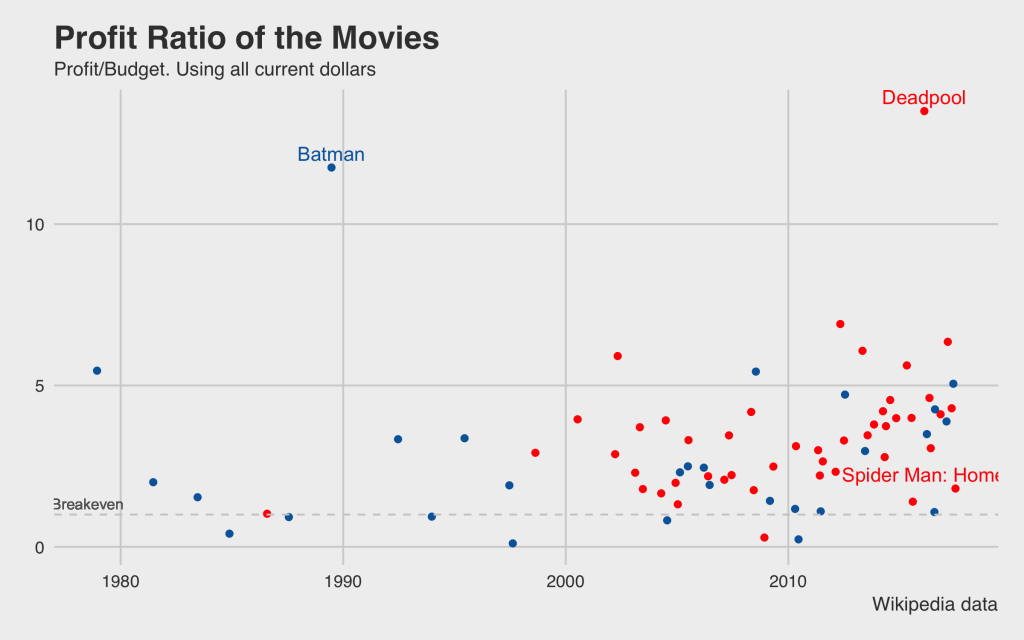Surprise, surprise. Deadpool had the budget of close to \$60M, yet it collected more than \$783M from the box office. That’s a profit to budget ratio of an impressive 13.5. For the DC universe, the Batman with Michael Keaton as Batman and Jack Nicholson as the Joker, released in 1989, grossed more than \$411M with the budget of \$35M. That’s impressive! And, ironically enough, Michael Keaton is the main villain of the new Spider-man movie, which is very close the break-even line.

Do you see any other trends that I missed?

# Complete Script

 ```1 2 3 4 5 6 7 8 9 10 11 12 13 14 15 16 17 18 19 20 21 22 23 24 25 26 27 28 29 30 31 32 33 34 35 36 37 38 39 40 41 42 43 44 45 46 47 48 49 50 51 52 53 54 55 56 57 58 59 60 61 62 63 64 65 66 67 68 69 70 71 72 73 74 75 76 77 78 79 ``` ```knitr::opts_chunk\$set(echo = TRUE) # To get data from Wikipedia library(rvest) # To manipulate data library(dplyr) # To plot library(ggplot2) # Make plots look good library(ggthemes) # Apply lables library(directlabels) # Better labeling library(scales) marvel_movies_data <- read_html('https://en.wikipedia.org/wiki/List_of_films_based_on_Marvel_Comics') %>% html_node(xpath = '//*[@id="mw-content-text"]/div/table') %>% html_table(fill = TRUE, trim = TRUE) %>% setNames(., nm = paste0("X", 1:8)) %>% slice(4:n()-2) %>% mutate_at(.cols = 4:8, .funs = funs(as.numeric(gsub(pattern = '[\$,]', replacement = '', x = .)))) %>% mutate(release_date = as.Date(X3, format = '%B %d, %Y'), movie = X1, universe = 'marvel', budget = X4*10^6, opening_wkend = X5, worldwide_gross = X8) dc_movies_data <- read_html('https://en.wikipedia.org/wiki/List_of_films_based_on_DC_Comics') %>% html_node(xpath = '//*[@id="mw-content-text"]/div/table') %>% html_table(fill = TRUE, trim = TRUE) %>% setNames(., nm = paste0("X", 1:7)) %>% slice(3:n()-1) %>% mutate_at(.cols = 4:7, .funs = funs(as.numeric(gsub(pattern = '[\$,]', replacement = '', x = .)))) %>% mutate(release_date = as.Date(X3, format = '%B %d, %Y'), movie = X1, universe = 'dc', budget = X4*10^6, opening_wkend = X5, worldwide_gross = X7) marvel_dc <- bind_rows(select(marvel_movies_data, universe, movie, release_date, budget, opening_wkend, worldwide_gross), select(dc_movies_data, universe, movie, release_date, budget, opening_wkend, worldwide_gross)) %>% mutate(profit = worldwide_gross - budget, profit_ratio = worldwide_gross/budget)   highest_profit_ratio <- group_by(marvel_dc, universe) %>% top_n( n = 1, wt = profit_ratio) %>% bind_rows(., filter(marvel_dc, movie == 'Spider Man: Homecoming')) highest_grossing <- group_by(marvel_dc, universe) %>% top_n( n = 1, wt = worldwide_gross) %>% bind_rows(., filter(marvel_dc, movie == 'Spider Man: Homecoming')) most_expensive <- group_by(marvel_dc, universe) %>% top_n( n = 1, wt = budget) %>% bind_rows(., filter(marvel_dc, movie == 'Spider Man: Homecoming')) most_profitable <- group_by(marvel_dc, universe) %>% top_n( n = 1, wt = profit) %>% bind_rows(., filter(marvel_dc, movie == 'Spider Man: Homecoming')) g <- ggplot(data = marvel_dc, aes(x = release_date, y = worldwide_gross, group = universe, color = universe)) + geom_point() + scale_y_continuous(labels = dollar) + theme_fivethirtyeight() g <- g + ylab("Worldwide Gross") + xlab(label = "Release Date") + scale_color_manual(values = c("dc" = "#0066A9", "marvel" = "red")) g <- g + geom_text(data = highest_grossing, aes(x = release_date, y = worldwide_gross, label = movie, color = universe), vjust = -0.5, show.legend = FALSE) g <- g + labs(title = "Worldwide Gross at the Box Office", subtitle = "All current dollars", caption = "Wikipedia data") g <- g + theme(legend.position = "none") g #g <- g + geom_smooth(se = FALSE, size = 0.4, span = 0.5) #ggsave(filename = "Worldwide-Gross-at-the-Box-Office-marvel-vs-dc.png", plot = g, width = 8, height = 5) g <- ggplot(data = marvel_dc, aes(x = release_date, y = budget, group = universe, color = universe)) + geom_point() + scale_y_continuous(labels = dollar) + theme_fivethirtyeight() g <- g + scale_color_manual(values = c("dc" = "#0066A9", "marvel" = "red")) g <- g + geom_text(data = most_expensive, aes(x = release_date, y = budget, label = movie, color = universe), vjust = -0.5, show.legend = FALSE) g <- g + labs(title = "Budget of the Movies", subtitle = "All current dollars", caption = "Wikipedia data") g <- g + theme(legend.position = "none") g #g <- g + geom_smooth(se = FALSE, size = 0.4, span = 0.5) #ggsave(filename = "budget-movies-marvel-vs-dc.png", plot = g, width = 8, height = 5) g <- ggplot(data = marvel_dc, aes(x = budget, y = worldwide_gross, group = universe, color = universe)) + geom_point() + scale_y_continuous(labels = dollar) + scale_x_continuous(labels = dollar) + theme_fivethirtyeight() g <- g + scale_color_manual(values = c("dc" = "#0066A9", "marvel" = "red")) #g <- g + geom_text(data = most_expensive, aes(x = release_date, y = budget, label = movie, color = universe), vjust = -0.5, show.legend = FALSE) g <- g + labs(title = "Budget and Worldwide Gross", subtitle = "All current dollars", caption = "Wikipedia data") g <- g + theme(legend.position = "none") g #g <- g + geom_smooth(se = FALSE, size = 0.4, span = 0.5) #ggsave(filename = "budget-worldwide-gross-movies-marvel-vs-dc.png", plot = g, width = 8, height = 5) g <- ggplot(data = marvel_dc, aes(x = release_date, y = profit, group = universe, color = universe)) + geom_point() + scale_y_continuous(labels = dollar) + theme_fivethirtyeight() g <- g + scale_color_manual(values = c("dc" = "#0066A9", "marvel" = "red")) g <- g + geom_text(data = most_profitable, aes(x = release_date, y = profit, label = movie, color = universe), vjust = -0.5, show.legend = FALSE) g <- g + labs(title = "Profit from the Movies", subtitle = "All current dollars", caption = "Wikipedia data") g <- g + theme(legend.position = "none") g #g <- g + geom_smooth(se = FALSE, size = 0.4, span = 0.5) #ggsave(filename = "profit-movies-marvel-vs-dc.png", plot = g, width = 8, height = 5) g <- ggplot(data = marvel_dc, aes(x = release_date, y = profit_ratio, group = universe, color = universe)) + geom_point() + scale_y_continuous() + theme_fivethirtyeight() g <- g + scale_color_manual(values = c("dc" = "#0066A9", "marvel" = "red")) g <- g + geom_text(data = highest_profit_ratio, aes(x = release_date, y = profit_ratio, label = movie, color = universe), vjust = -0.5, show.legend = FALSE) g <- g + labs(title = "Profit Ratio of the Movies", subtitle = "Profit/Budget. Using all current dollars", caption = "Wikipedia data") g <- g + theme(legend.position = "none") g <- g + geom_hline(yintercept = 1, color = "grey80", linetype = 2) + geom_text(x = 1980, y = 1, label = "Breakeven", hjust = -0.45, vjust = -0.5, color = "grey40", size = rel(3)) g #g <- g + geom_smooth(se = FALSE, size = 0.4, span = 0.5) #ggsave(filename = "profit-ratio-budget-profit-movies-marvel-vs-dc.png", plot = g, width = 8, height = 5)```

The post DC vs Marvel Comic Universe: which movies are doing better? appeared first on nandeshwar.info.

R-bloggers.com offers daily e-mail updates about R news and tutorials about learning R and many other topics. Click here if you're looking to post or find an R/data-science job.
Want to share your content on R-bloggers? click here if you have a blog, or here if you don't.